Comment on page

# Java 容器

## 一、概览

Java容器大致可以分为Set、List、Queue（这三种都属于 Collection）和Map四种体系。
• Set代表无序、不可重复的集合。（Collection）
• List代表有序、可重复的集合。（Collection）
• Queue代表一种队列集合实现。（Collection）
• Map则代表具有映射关系的集合。

### Collection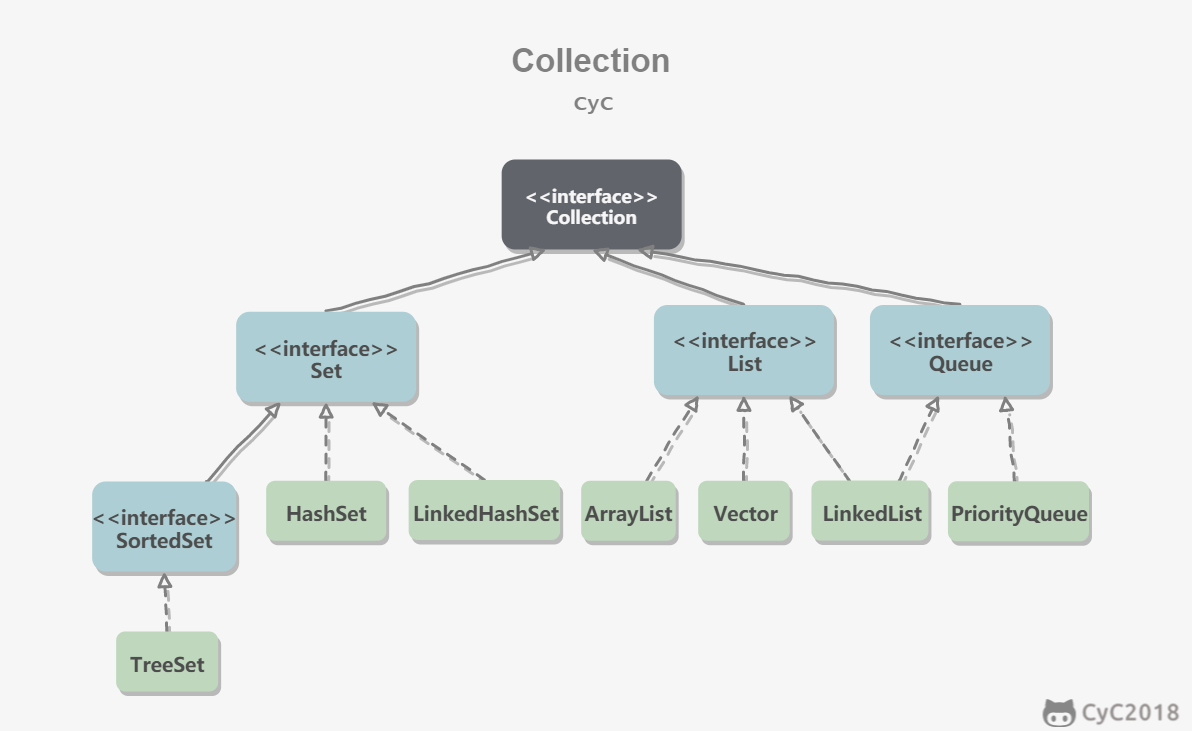#### 1. Set

• TreeSet：基于红黑树实现，支持有序性操作，例如根据一个范围查找元素的操作。但是查找效率不如 HashSet，HashSet 查找的时间复杂度为 O(1)，TreeSet 则为 O(logN)。
• HashSet：基于哈希表实现，支持快速查找，但不支持有序性操作。并且失去了元素的插入顺序信息，也就是说使用 Iterator 遍历 HashSet 得到的结果是不确定的。

#### 2. List

• ArrayList：基于动态数组实现，支持随机访问。
• Vector：和 ArrayList 类似，但它是线程安全的。

#### 3. Queue

• PriorityQueue：基于堆结构实现，可以用它来实现优先队列。

### Map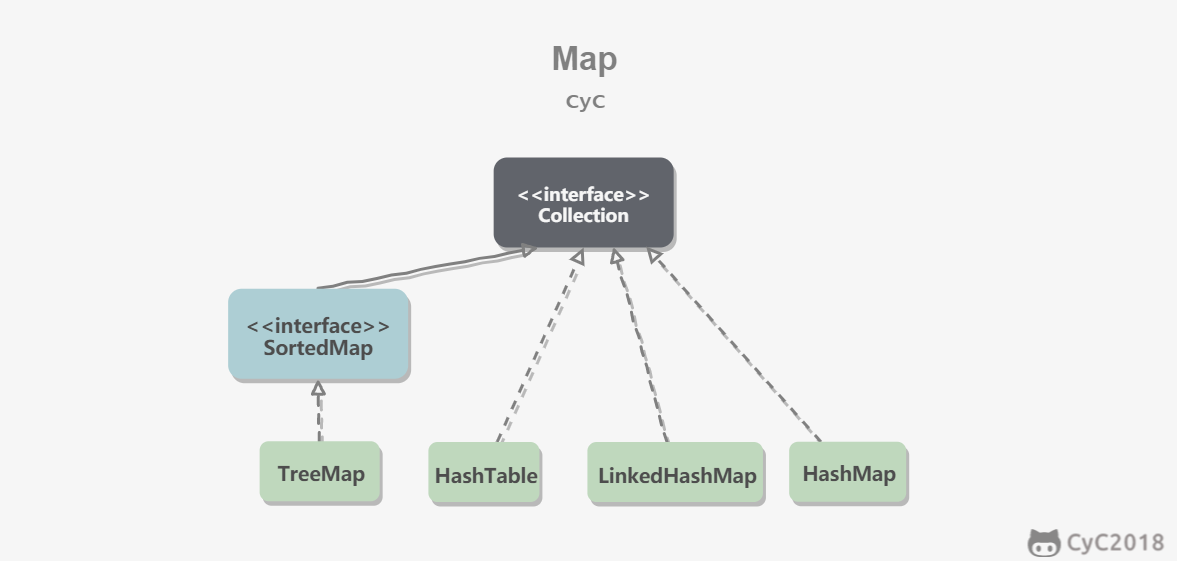• TreeMap：基于红黑树实现。
• HashMap：基于哈希表实现。
• HashTable：和 HashMap 类似，但它是线程安全的，这意味着同一时刻多个线程同时写入 HashTable 不会导致数据不一致。它是遗留类，不应该去使用它，应该使用 ConcurrentHashMap 来支持线程安全，ConcurrentHashMap 的效率会更高，因为 ConcurrentHashMap 引入了分段锁。

## 二、容器中的设计模式

### 迭代器模式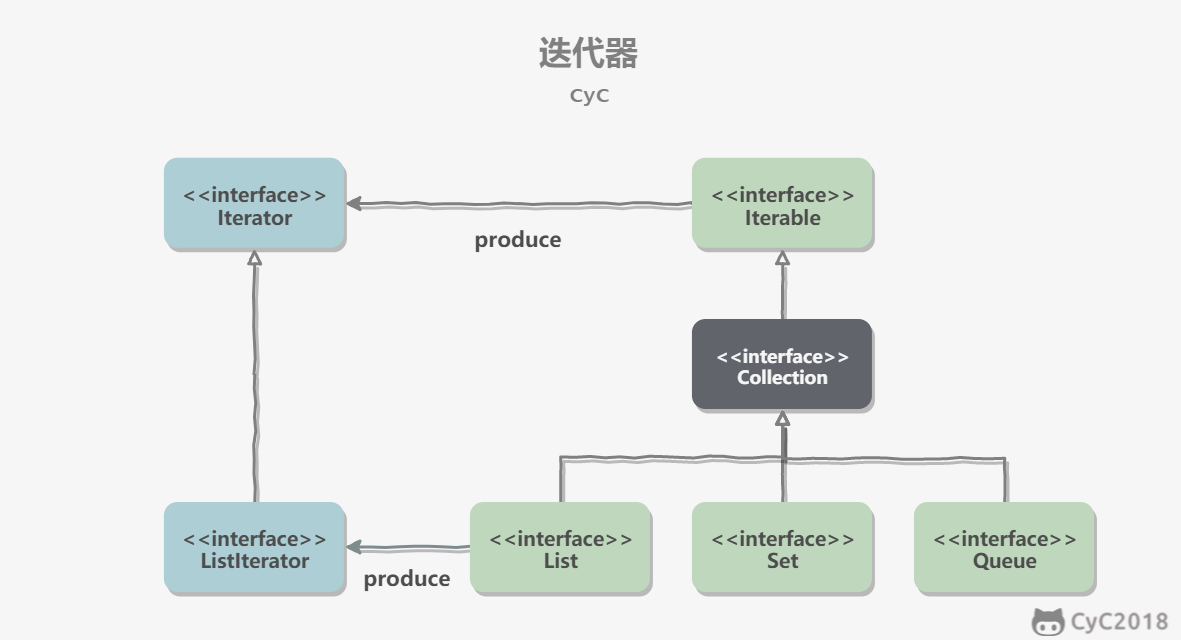Collection 继承了 Iterable 接口，其中的 iterator() 方法能够产生一个 Iterator 对象，通过这个对象就可以迭代遍历 Collection 中的元素。

List<String> list = new ArrayList<>();
for (String item : list) {
System.out.println(item);
}

### 适配器模式

java.util.Arrays#asList() 可以把数组类型转换为 List 类型。
@SafeVarargs
public static <T> List<T> asList(T... a)

Integer[] arr = {1, 2, 3};
List list = Arrays.asList(arr);

List list = Arrays.asList(1, 2, 3);

## 三、源码分析

### ArrayList

#### 1. 概览

public class ArrayList<E> extends AbstractList<E>
implements List<E>, RandomAccess, Cloneable, java.io.Serializable

private static final int DEFAULT_CAPACITY = 10;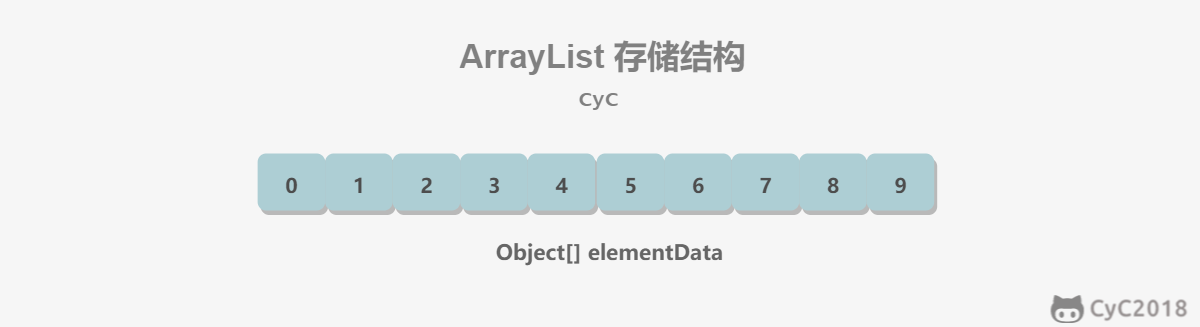#### 2. 扩容

`List<Integer> list = new ArrayList<>(20);`
ensureCapacityInternal(size + 1); // Increments modCount!!
elementData[size++] = e;
return true;
}
private void ensureCapacityInternal(int minCapacity) {
if (elementData == DEFAULTCAPACITY_EMPTY_ELEMENTDATA) {
minCapacity = Math.max(DEFAULT_CAPACITY, minCapacity);
}
ensureExplicitCapacity(minCapacity);
}
private void ensureExplicitCapacity(int minCapacity) {
modCount++;
// overflow-conscious code
if (minCapacity - elementData.length > 0)
grow(minCapacity);
}
private void grow(int minCapacity) {
// overflow-conscious code
int oldCapacity = elementData.length;
int newCapacity = oldCapacity + (oldCapacity >> 1);
if (newCapacity - minCapacity < 0)
newCapacity = minCapacity;
if (newCapacity - MAX_ARRAY_SIZE > 0)
newCapacity = hugeCapacity(minCapacity);
// minCapacity is usually close to size, so this is a win:
elementData = Arrays.copyOf(elementData, newCapacity);
}

#### 3. 删除元素

public E remove(int index) {
rangeCheck(index);
modCount++;
E oldValue = elementData(index);
int numMoved = size - index - 1;
if (numMoved > 0)
System.arraycopy(elementData, index+1, elementData, index, numMoved);
elementData[--size] = null; // clear to let GC do its work
return oldValue;
}

#### 4. 序列化

ArrayList 基于数组实现，并且具有动态扩容特性，因此保存元素的数组不一定都会被使用，那么就没必要全部进行序列化。

transient Object[] elementData; // non-private to simplify nested class access
ArrayList 实现了 writeObject() 和 readObject() 来控制只序列化数组中有元素填充那部分内容。
throws java.io.IOException, ClassNotFoundException {
elementData = EMPTY_ELEMENTDATA;
// Read in size, and any hidden stuff
if (size > 0) {
// be like clone(), allocate array based upon size not capacity
ensureCapacityInternal(size);
Object[] a = elementData;
// Read in all elements in the proper order.
for (int i=0; i<size; i++) {
}
}
}
private void writeObject(java.io.ObjectOutputStream s)
throws java.io.IOException{
// Write out element count, and any hidden stuff
int expectedModCount = modCount;
s.defaultWriteObject();
// Write out size as capacity for behavioural compatibility with clone()
s.writeInt(size);
// Write out all elements in the proper order.
for (int i=0; i<size; i++) {
s.writeObject(elementData[i]);
}
if (modCount != expectedModCount) {
throw new ConcurrentModificationException();
}
}

writeObject() ：对象转换为字节序列。
ArrayList list = new ArrayList();
ObjectOutputStream oos = new ObjectOutputStream(new FileOutputStream(file));
oos.writeObject(list);

#### 5. Fail-Fast

modCount 用来记录 ArrayList 结构发生变化的次数。结构发生变化是指添加或者删除至少一个元素的所有操作，或者是调整内部数组的大小，仅仅只是设置元素的值不算结构发生变化。

### Vector

ArrayList + synchronized = Vector

#### 1. 同步

public synchronized boolean add(E e) {
modCount++;
ensureCapacityHelper(elementCount + 1);
elementData[elementCount++] = e;
return true;
}
public synchronized E get(int index) {
if (index >= elementCount)
throw new ArrayIndexOutOfBoundsException(index);
return elementData(index);
}

#### 2. 扩容

Vector 的构造函数可以传入 capacityIncrement 参数，它的作用是在扩容时使容量 capacity 增长 capacityIncrement。如果这个参数的值小于等于 0，扩容时每次都令 capacity 为原来的两倍。
public Vector(int initialCapacity, int capacityIncrement) {
super();
if (initialCapacity < 0)
throw new IllegalArgumentException("Illegal Capacity: "+
initialCapacity);
this.elementData = new Object[initialCapacity];
this.capacityIncrement = capacityIncrement;
}
private void grow(int minCapacity) {
// overflow-conscious code
int oldCapacity = elementData.length;
int newCapacity = oldCapacity + ((capacityIncrement > 0) ?
capacityIncrement : oldCapacity);
if (newCapacity - minCapacity < 0)
newCapacity = minCapacity;
if (newCapacity - MAX_ARRAY_SIZE > 0)
newCapacity = hugeCapacity(minCapacity);
elementData = Arrays.copyOf(elementData, newCapacity);
}

public Vector(int initialCapacity) {
this(initialCapacity, 0);
}
public Vector() {
this(10);
}

#### 3. 与 ArrayList 的比较

• Vector 是同步的，因此开销就比 ArrayList 要大，访问速度更慢。最好使用 ArrayList 而不是 Vector，因为同步操作完全可以由程序员自己来控制。
• Vector 每次扩容请求其大小的 2 倍（也可以通过构造函数设置增长的容量），而 ArrayList 是 1.5 倍。

#### 4. 替代方案

List<String> list = new ArrayList<>();
List<String> synList = Collections.synchronizedList(list);

List<String> list = new CopyOnWriteArrayList<>();

### CopyOnWriteArrayList

#### 1. 读写分离

final ReentrantLock lock = this.lock;
lock.lock();
try {
Object[] elements = getArray();
int len = elements.length;
Object[] newElements = Arrays.copyOf(elements, len + 1);
newElements[len] = e;
setArray(newElements);
return true;
} finally {
lock.unlock();
}
}
final void setArray(Object[] a) {
array = a;
}
@SuppressWarnings("unchecked")
private E get(Object[] a, int index) {
return (E) a[index];
}

#### 2. 适用场景

CopyOnWriteArrayList 在写操作的同时允许读操作，大大提高了读操作的性能，因此很适合读多写少的应用场景。

• 内存占用：在写操作时需要复制一个新的数组，使得内存占用为原来的两倍左右；
• 数据不一致：读操作不能读取实时性的数据，因为部分写操作的数据还未同步到读数组中。

#### 1. 概览

private static class Node<E> {
E item;
Node<E> next;
Node<E> prev;
}

transient Node<E> first;
transient Node<E> last;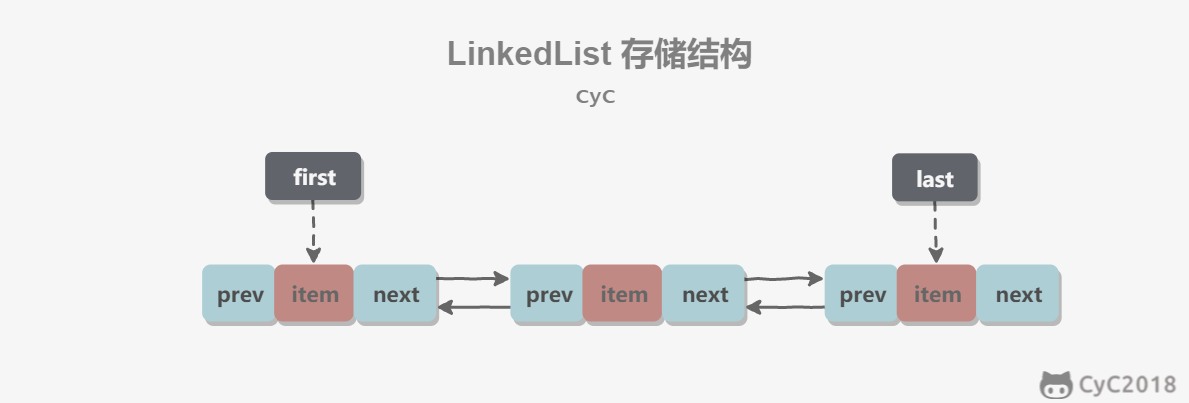#### 2. 与 ArrayList 的比较

• 数组支持随机访问，但插入删除的代价很高，需要移动大量元素；
• 链表不支持随机访问，但插入删除只需要改变指针。

### HashMap⭐️

#### 1. 存储结构

HashMap 使用拉链法来解决冲突，同一个链表中存放哈希值和散列桶取模运算结果相同的 Entry。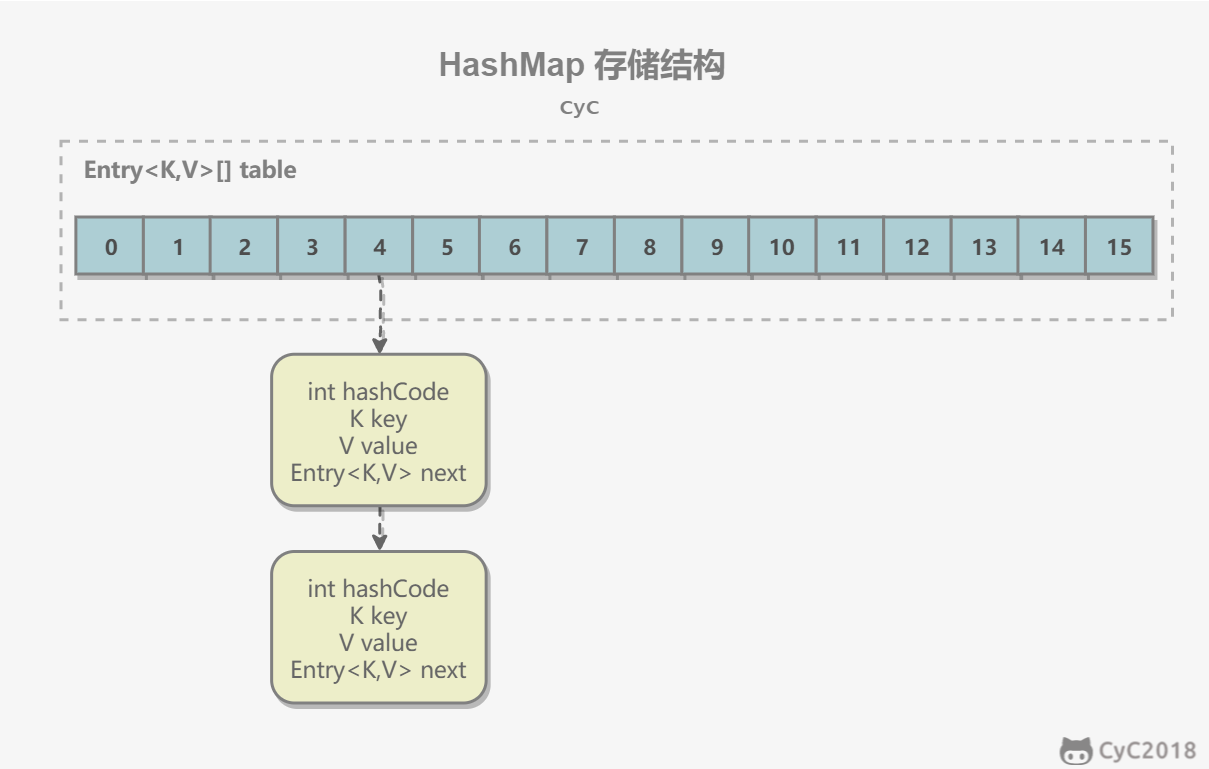transient Entry[] table;
static class Entry<K,V> implements Map.Entry<K,V> {
final K key;
V value;
Entry<K,V> next;
int hash;
Entry(int h, K k, V v, Entry<K,V> n) {
value = v;
next = n;
key = k;
hash = h;
}
public final K getKey() {
return key;
}
public final V getValue() {
return value;
}
public final V setValue(V newValue) {
V oldValue = value;
value = newValue;
return oldValue;
}
public final boolean equals(Object o) {
if (!(o instanceof Map.Entry))
return false;
Map.Entry e = (Map.Entry)o;
Object k1 = getKey();
Object k2 = e.getKey();
if (k1 == k2 || (k1 != null && k1.equals(k2))) {
Object v1 = getValue();
Object v2 = e.getValue();
if (v1 == v2 || (v1 != null && v1.equals(v2)))
return true;
}
return false;
}
public final int hashCode() {
return Objects.hashCode(getKey()) ^ Objects.hashCode(getValue());
}
public final String toString() {
return getKey() + "=" + getValue();
}
}

#### 2. 拉链法的工作原理

HashMap<String, String> map = new HashMap<>();
map.put("K1", "V1");
map.put("K2", "V2");
map.put("K3", "V3");
• 新建一个 HashMap，默认大小为 16；
• 插入 <K1,V1> 键值对，先计算 K1 的 hashCode 为 115，使用除留余数法得到所在的桶下标 115%16=3。
• 插入 <K2,V2> 键值对，先计算 K2 的 hashCode 为 118，使用除留余数法得到所在的桶下标 118%16=6。
• 插入 <K3,V3> 键值对，先计算 K3 的 hashCode 为 118，使用除留余数法得到所在的桶下标 118%16=6，插在 <K2,V2> 前面。

• 计算键值对所在的桶；
• 在链表上顺序查找，时间复杂度显然和链表的长度成正比。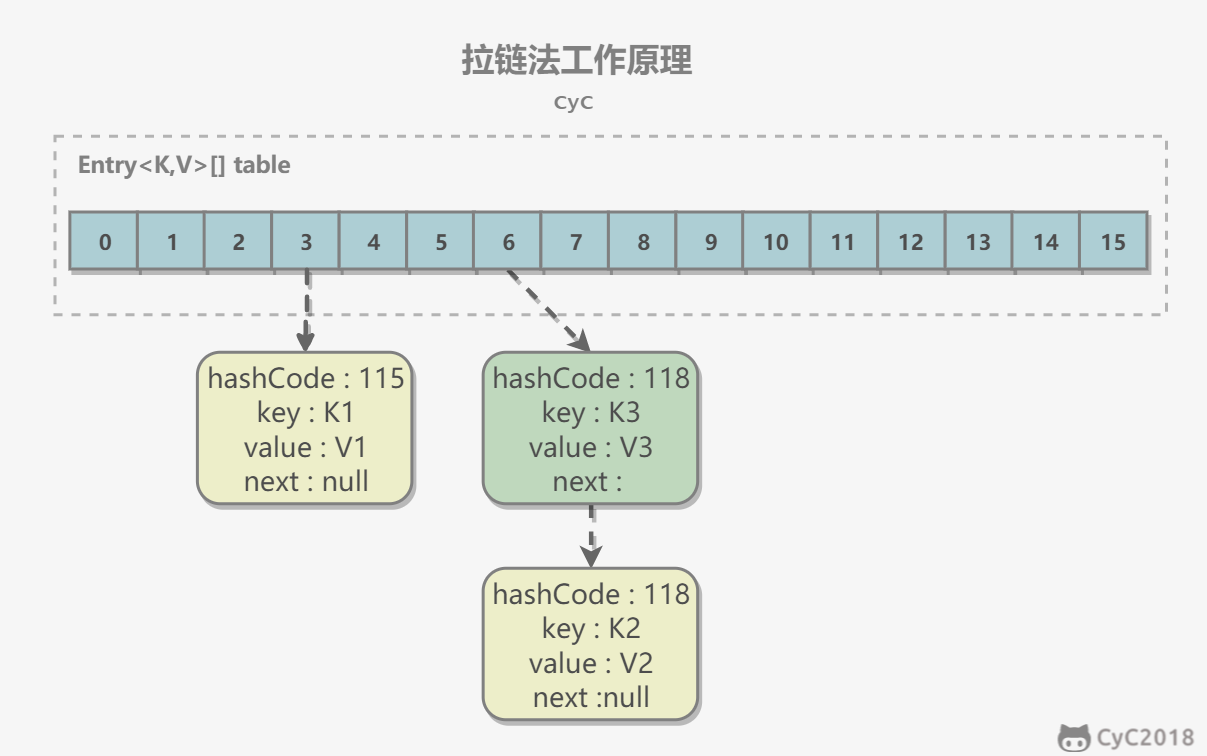#### 3. put 操作

① 如果定位到的数组位置没有元素，就直接插入。
② 如果定位到的数组位置有元素，遍历以这个元素为头结点的链表，依次和插入的 `key` 比较，如果 `key` 相同就直接覆盖，不同就采用头插法插入元素。
public V put(K key, V value) {
if (table == EMPTY_TABLE) {
inflateTable(threshold);
}
// 键为 null 单独处理
if (key == null)
return putForNullKey(value);
int hash = hash(key);
// 确定桶下标
int i = indexFor(hash, table.length);
// 先找出是否已经存在键为 key 的键值对，如果存在的话就更新这个键值对的值为 value
for (Entry<K,V> e = table[i]; e != null; e = e.next) {
Object k;
if (e.hash == hash && ((k = e.key) == key || key.equals(k))) {
V oldValue = e.value;
e.value = value;
e.recordAccess(this);
return oldValue;
}
}
modCount++;
// 插入新键值对
return null;
}
HashMap 允许插入键为 null 的键值对。但是因为无法调用 null 的 hashCode() 方法，也就无法确定该键值对的桶下标，只能通过强制指定一个桶下标来存放。
HashMap 使用第 0 个桶存放键为 null 的键值对。
private V putForNullKey(V value) {
for (Entry<K,V> e = table; e != null; e = e.next) {
if (e.key == null) {
V oldValue = e.value;
e.value = value;
e.recordAccess(this);
return oldValue;
}
}
modCount++;
return null;
}

void addEntry(int hash, K key, V value, int bucketIndex) {
if ((size >= threshold) && (null != table[bucketIndex])) {
resize(2 * table.length);
hash = (null != key) ? hash(key) : 0;
bucketIndex = indexFor(hash, table.length);
}
createEntry(hash, key, value, bucketIndex);
}
void createEntry(int hash, K key, V value, int bucketIndex) {
Entry<K,V> e = table[bucketIndex];
// 头插法，链表头部指向新的键值对
table[bucketIndex] = new Entry<>(hash, key, value, e);
size++;
}
Entry(int h, K k, V v, Entry<K,V> n) {
value = v;
next = n;
key = k;
hash = h;
}

#### 4. 确定桶下标

int hash = hash(key);
int i = indexFor(hash, table.length);
4.1 计算 hash 值
final int hash(Object k) {
int h = hashSeed;
if (0 != h && k instanceof String) {
return sun.misc.Hashing.stringHash32((String) k);
}
h ^= k.hashCode();
// This function ensures that hashCodes that differ only by
// constant multiples at each bit position have a bounded
// number of collisions (approximately 8 at default load factor).
h ^= (h >>> 20) ^ (h >>> 12);
return h ^ (h >>> 7) ^ (h >>> 4);
}
public final int hashCode() {
return Objects.hashCode(key) ^ Objects.hashCode(value);
}
4.2 取模

x : 00010000
x-1 : 00001111

y : 10110010
x-1 : 00001111
y&(x-1) : 00000010

y : 10110010
x : 00010000
y%x : 00000010

static int indexFor(int h, int length) {
return h & (length-1);
}

#### 5. 扩容-基本原理

 参数 含义 capacity table 的容量大小，默认为 16。需要注意的是 capacity 必须保证为 2 的 n 次方。 size 键值对数量。 threshold size 的临界值，当 size 大于等于 threshold 就必须进行扩容操作。 loadFactor 装载因子，table 能够使用的比例，threshold = (int)(capacity* loadFactor)。

static final int DEFAULT_INITIAL_CAPACITY = 16;
static final int MAXIMUM_CAPACITY = 1 << 30;
static final float DEFAULT_LOAD_FACTOR = 0.75f;
transient Entry[] table;
transient int size;
int threshold;
transient int modCount;

void addEntry(int hash, K key, V value, int bucketIndex) {
Entry<K,V> e = table[bucketIndex];
table[bucketIndex] = new Entry<>(hash, key, value, e);
if (size++ >= threshold)
resize(2 * table.length);
}

void resize(int newCapacity) {
Entry[] oldTable = table;
int oldCapacity = oldTable.length;
if (oldCapacity == MAXIMUM_CAPACITY) {
threshold = Integer.MAX_VALUE;
return;
}
Entry[] newTable = new Entry[newCapacity];
transfer(newTable);
table = newTable;
}
void transfer(Entry[] newTable) {
Entry[] src = table;
int newCapacity = newTable.length;
for (int j = 0; j < src.length; j++) {
Entry<K,V> e = src[j];
if (e != null) {
src[j] = null;
do {
Entry<K,V> next = e.next;
int i = indexFor(e.hash, newCapacity);
e.next = newTable[i];
newTable[i] = e;
e = next;
} while (e != null);
}
}
}

#### 6. 扩容-重新计算桶下标

capacity : 00010000
new capacity : 00100000

• 为 0，那么 hash%00010000 = hash%00100000，桶位置和原来一致；
• 为 1，hash%00010000 = hash%00100000 + 16，桶位置是原位置 + 16。

#### 7. 计算数组容量

HashMap 构造函数允许用户传入的容量不是 2 的 n 次方，因为它可以自动地将传入的容量转换为 2 的 n 次方。

mask+1 是大于原始数字的最小的 2 的 n 次方。
num 10010000

static final int tableSizeFor(int cap) {
int n = cap - 1;
n |= n >>> 1;
n |= n >>> 2;
n |= n >>> 4;
n |= n >>> 8;
n |= n >>> 16;
return (n < 0) ? 1 : (n >= MAXIMUM_CAPACITY) ? MAXIMUM_CAPACITY : n + 1;
}

#### 9. 与 Hashtable 的比较

• Hashtable 使用 synchronized 来进行同步。
• HashMap 可以插入键为 null 的 Entry。
• HashMap 的迭代器是 fail-fast 迭代器。
• HashMap 不能保证随着时间的推移 Map 中的元素次序是不变的。

#### 11. get 操作

int hash = (key == null) ? 0 : hash(key);
for (Entry<K,V> e = table[indexFor(hash, table.length)];e != null;e = e.next) {
Object k;
if (e.hash == hash &&
((k = e.key) == key || (key != null && key.equals(